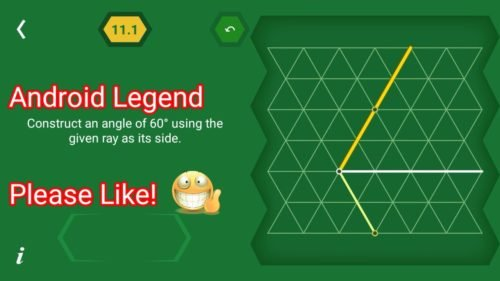# Pythagorea 60 degree Level 11.1 11.2 11.3 11.4 11.5 11.6 11.7 11.8 11.9 11.10 11.11 Solution/AnswersPythagorea 60 degrees is android/iOS app developed by Horis International Limited. This game is mostly focused on geometric puzzles and construction. The workspace is divided into triangular grids lines aligned 60° to each other, that’s why this game names as pythagorea 60°. You should know all the basic Math operations. All lines and shapes are drawn on a grid whose cells are equilateral triangles. Most of the game levels can be answered using natural intuition and bye some basic laws of geometry.

You have to connect points on the grid using straight lines to construct an element, you can even use intersection points to draw. some levels are very easy some are of medium difficulty and some are very hard to solve, that’s why I am providing solutions to all the problems.

If you are here for levels other than ‘Angles’ Go to directory of all other levels at : http://www.puzzlegamemaster.com/pythagorea-60-complete/

• Pythagorea 60° Level 11.1: Construct an angle of 60 using the given ray as its side.• Pythagorea 60° Level 11.2: Construct an angle of 60 using the given ray as its side.• Pythagorea 60° Level 11.3: Construct an angle of 60 using the given ray as its side.• Pythagorea 60° Level 11.4: Construct an angle of 120 using the given ray as its side.• Pythagorea 60° Level 11.5: Construct an angle of 30 using the given ray as its side.• Pythagorea 60° Level 11.6: Construct an angle of 30 using the given ray as its side.• Pythagorea 60° Level 11.7: Construct an angle of 150 using the given ray as its side.• Pythagorea 60° Level 11.8: Reflect the angle across the given line AB.• Pythagorea 60° Level 11.9: Reflect the angle across the given line AB.• Pythagorea 60° Level 11.10: Construct a rectangle whose diagonals intersect at 60 given a side.• Pythagorea 60° Level 11.11: Construct a rectangle whose diagonals intersect at 60 given a side.Feel free to comment below if you have any doubts regarding the solutions. I will try to help you guys. All other levels are posted on this blog please visit them too.